Related Articles

# Control Signals Generation using Counter

• Last Updated : 19 May, 2021
• In various digital applications(For example : hardwired control unit) control signals are needed to start, execute and step various operations in a particular time sequence.
• For this control signals are required and for the generation of control signals, a counter circuit is designed whose outputs are connected to a decoder. The decoder provides the required control signal.
• The counter can be synchronous or asynchronous.
• The procedure for designing the counter is the same refer (this).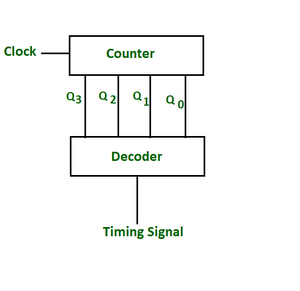Block diagram for control signal generation

The design of control signal can be understood by considering the example.
Example – Generate a control signal which can deliver the following pulse train. The pulse train will repeat after 7 pulses.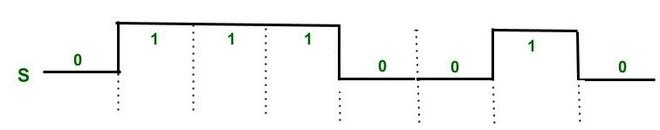Control Signal

Here we need to generate a control signal (say S) which can generate periodic pulse train of 0111001 and then repeats. The pulse train repeats after 7 seven pulses. Therefore, mod -7 counter is needed. The outputs of Mod-7 counter will be connected to a decoder circuit. For this a mod –7 counter is to be designed. Three T flips are required(because we need to count up to 000 to 110, therefore 3 bits are needed).

Design for mod-7 counter –
Here Q is previous state and Q* is next state.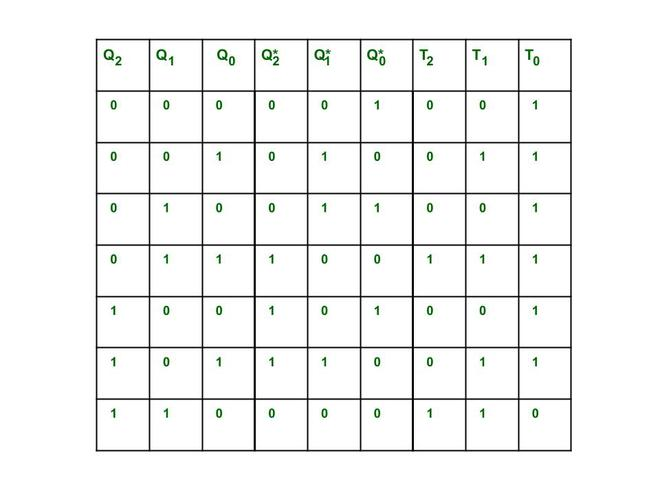Circuit excitation table for mod- 7 Counter

The expressions for inputs of T flip-flops obtained from the K – maps shown below.The truth table for the decoder is obtained by observing of the given timing sequence(0111001). The simplified expression for the output S of decoder is obtained using the K-map. The not used counts are considered as don’t care.
Each counting sequence is mapped to one control signal bit.
The combinational logic of decoder can be found by solving K map.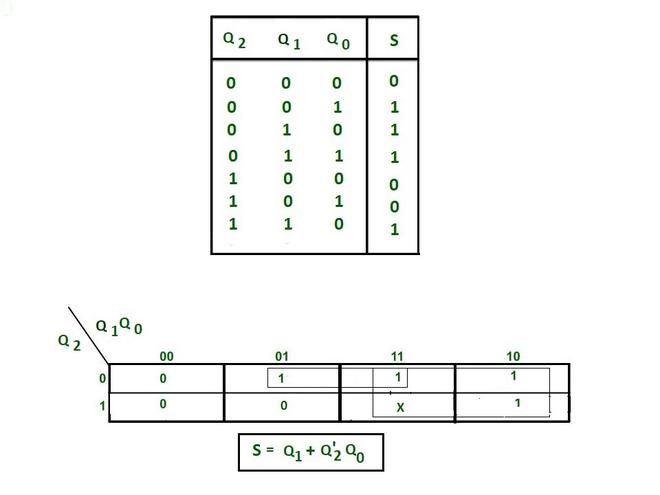Simplified expression for decoder circuit is S= Q1+ Q’2Q0.
Example — When we are in state Q2 =0 Q1= 0 Q0 = 0, then the value of S = 0+0.1= 0.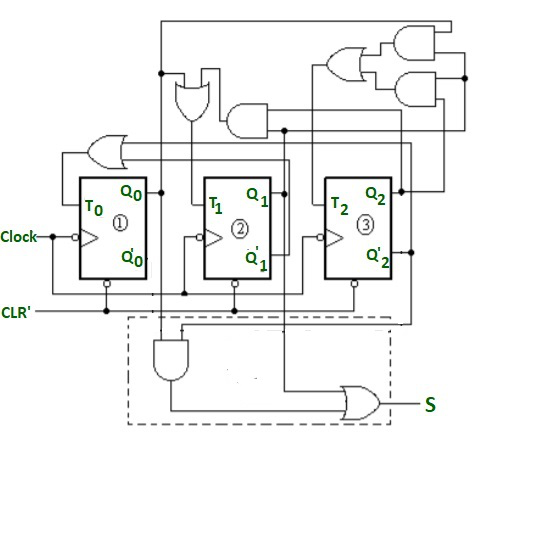Complete logic diagram with Decoder.

The decoder circuit generates output after every clock(-ve edge triggered) pulse. The output i.e S will be act as Control signal for other circuitry.

The generation of a control signal takes place after each negative edge clock and timing diagram for such control signal may also be drawn as shown below. Each counter state is used for generating one control signal.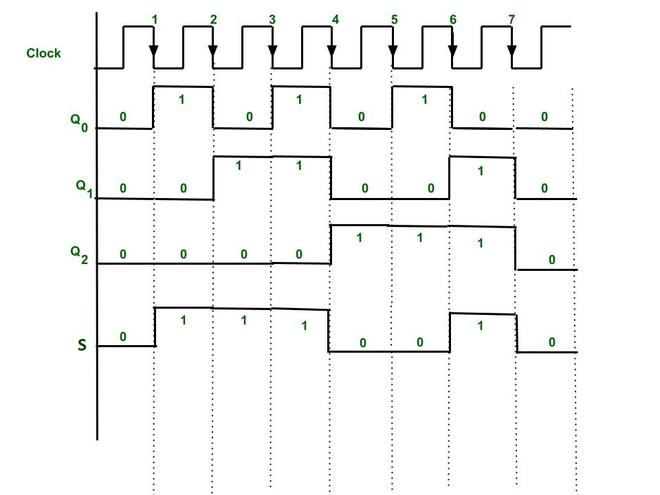Timing diagram for Control signal S generation by counter

Attention reader! Don’t stop learning now.  Practice GATE exam well before the actual exam with the subject-wise and overall quizzes available in GATE Test Series Course.

Learn all GATE CS concepts with Free Live Classes on our youtube channel.

My Personal Notes arrow_drop_up